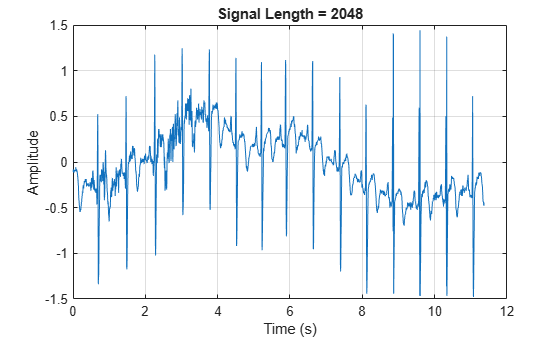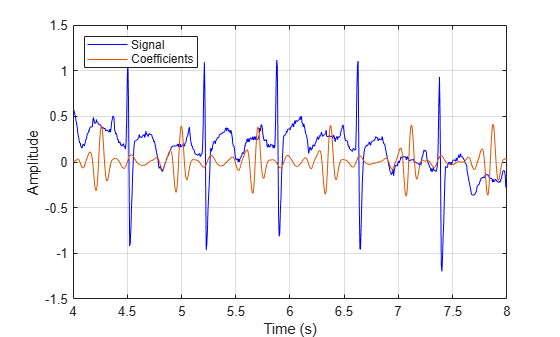# modwtmra

Multiresolution analysis based on MODWT

## Syntax

``mra = modwtmra(w)``
``mra = modwtmra(w,wname) ``
``mra = modwtmra(w,Lo,Hi)``
``mra = modwtmra(___,'reflection')``

## Description

example

````mra = modwtmra(w)` returns the multiresolution analysis (MRA) of the maximal overlap discrete wavelet transform (MODWT) matrix, `w`. The MODWT matrix, `w`, is the output of the `modwt` function. By default, `modwtmra` assumes that you obtained `w` using the `'sym4'` wavelet with periodic boundary handling.```

example

````mra = modwtmra(w,wname) ` constructs the MRA using the wavelet corresponding to `wname`. The `wname` wavelet must be the same wavelet used to obtain the MODWT. ```

example

````mra = modwtmra(w,Lo,Hi)` constructs the MRA using the scaling filter `Lo` and wavelet filter `Hi`. The `Lo` and `Hi` filters must be the same filters used to obtain the MODWT.```

example

````mra = modwtmra(___,'reflection')` uses the reflection boundary condition in the construction of the MRA using any of the arguments from previous syntaxes. If you specify `'reflection'`, `modwtmra` assumes that the column dimension of `w` is even and equals twice the length of the original signal.You must enter the entire character vector `'reflection'`. If you added a wavelet named `'reflection'` using the wavelet manager, you must rename that wavelet prior to using this option. `'reflection'` may be placed in any position in the input argument list after `x`. By default, `modwtmra` uses periodic extension at the boundary.```

## Examples

collapse all

Obtain the MODWTMRA of a simple time-series signal and demonstrate perfect reconstruction.

Create a time-series signal

```t = 1:10; x = sin(2*pi*200*t);```

Obtain the MODWT and the MODWTMRA and sum the MODWTMRA rows.

```m = modwt(x); mra = modwtmra(m); xrec = sum(mra);```

Use the maximum of the absolute values to show that the difference between the original signal and the reconstruction is extremely small. The largest absolute value is on the order of $1{0}^{-25}$, which demonstrates perfect reconstruction.

`max(abs(x-xrec))`
```ans = 5.5738e-25 ```

Construct an MRA of an ECG signal down to level four using the `db2` wavelet. The data are taken from Percival & Walden (2000), p.125 (data originally provided by William Constantine and Per Reinhall, University of Washington). The sampling frequency for the ECG signal is 180 hertz.

```load wecg; lev = 4; wtecg = modwt(wecg,'db2',lev); mra = modwtmra(wtecg,'db2');```

Plot the ECG waveform and the MRA.

```t = (0:numel(wecg)-1)/180; subplot(6,1,1) plot(t,wecg) for kk = 2:lev+2 subplot(6,1,kk) plot(t,mra(kk-1,:)) end xlabel('Time (s)') set(gcf,'Position',[0 0 500 700])```Construct a multiresolution analysis for the Southern Oscillation Index data. The sampling period is one day. Plot the level eight details corresponding to a scale of ${2}^{8}$ days. The details at this scale capture oscillations on a scale of approximately one year.

```load soi wtsoi = modwt(soi); mrasoi = modwtmra(wtsoi); plot(mrasoi(8,:)) title('Level 8 Details')```Obtain the MRA for the Deutsch Mark - U.S. Dollar exchange rate data using the minimum bandwidth scaling and wavelet filters with four coefficients.

```load DM_USD; Lo = [0.4801755, 0.8372545, 0.2269312, -0.1301477]; Hi = qmf(Lo); wdm = modwt(DM_USD,Lo,Hi); mra = modwtmra(wdm,Lo,Hi);```

Obtain the MRA for an ECG signal using `'reflection'` boundary handling. The data are taken from Percival & Walden (2000), p.125 (data originally provided by William Constantine and Per Reinhall, University of Washington).

```load wecg; wtecg = modwt(wecg,'reflection'); mra = modwtmra(wtecg,'reflection');```

Show that the number of columns in the MRA is equal to the number of elements in the original signal.

`isequal(size(mra,2),numel(wecg))`
```ans = logical 1 ```

Load the 23 channel EEG data `Espiga3` . The channels are arranged column-wise. The data is sampled at 200 Hz.

`load Espiga3`

Obtain the MRA of the multisignal.

```w = modwt(Espiga3); mra = modwtmra(w);```

This example demonstrates the differences between the functions MODWT and MODWTMRA. The MODWT partitions a signal's energy across detail coefficients and scaling coefficients. The MODWTMRA projects a signal onto wavelet subspaces and a scaling subspace.

Choose the `sym6` wavelet. Load and plot an electrocardiogram (ECG) signal. The sampling frequency for the ECG signal is 180 hertz. The data are taken from Percival and Walden (2000), p.125 (data originally provided by William Constantine and Per Reinhall, University of Washington).

```load wecg t = (0:numel(wecg)-1)/180; wv = 'sym6'; plot(t,wecg) grid on title(['Signal Length = ',num2str(numel(wecg))]) xlabel('Time (s)') ylabel('Amplitude')```Take the MODWT of the signal.

`wtecg = modwt(wecg,wv);`

The input data are samples of a function $f\left(x\right)$ evaluated at $N$-many time points. The function can be expressed as a linear combination of the scaling function $\varphi \left(x\right)$ and wavelet $\psi \left(x\right)$at varying scales and translations: $f\left(x\right)=\sum _{k=0}^{N-1}{c}_{k}\phantom{\rule{0.16666666666666666em}{0ex}}{2}^{-{J}_{0}/2}\varphi \left({2}^{-{J}_{0}}\phantom{\rule{0.16666666666666666em}{0ex}}x-k\right)+\sum _{j=1}^{{J}_{0}}{f}_{j}\left(x\right)$ where ${f}_{j}\left(x\right)=\sum _{k=0}^{N-1}{d}_{j,k}\phantom{\rule{0.16666666666666666em}{0ex}}{2}^{-j/2}\phantom{\rule{0.16666666666666666em}{0ex}}\psi \left({2}^{-j}x-k\right)$ and ${J}_{0}$ is the number of levels of wavelet decomposition. The first sum is the coarse scale approximation of the signal, and the ${f}_{j}\left(x\right)$ are the details at successive scales. MODWT returns the $N$-many coefficients $\left\{{c}_{k}\right\}$and the $\left({J}_{0}×N\right)$-many detail coefficients $\left\{{d}_{j,k}\right\}$ of the expansion. Each row in `wtecg` contains the coefficients at a different scale.

When taking the MODWT of a signal of length $N$, there are $\text{floor}\left({\mathrm{log}}_{2}\left(N\right)\right)$-many levels of decomposition (by default). Detail coefficients are produced at each level. Scaling coefficients are returned only for the final level. In this example, since $N=2048$, ${J}_{0}=\text{floor}\left(\mathrm{log}2\left(2048\right)\right)=11$ and the number of rows in `wtecg` is ${J}_{0}+1=11+1=12$.

The MODWT partitions the energy across the various scales and scaling coefficients: ${||X||}^{2}=\sum _{j=1}^{{J}_{0}}{||{W}_{j}||}^{2}+{||{V}_{{J}_{0}}||}^{2}$ where $X$ is the input data, ${W}_{j}$ are the detail coefficients at scale $j$, and ${V}_{{J}_{0}}$ are the final-level scaling coefficients.

Compute the energy at each scale, and evaluate their sum.

```energy_by_scales = sum(wtecg.^2,2); Levels = {'D1';'D2';'D3';'D4';'D5';'D6';'D7';'D8';'D9';'D10';'D11';'A11'}; energy_table = table(Levels,energy_by_scales); disp(energy_table)```
``` Levels energy_by_scales _______ ________________ {'D1' } 14.063 {'D2' } 20.612 {'D3' } 37.716 {'D4' } 25.123 {'D5' } 17.437 {'D6' } 8.9852 {'D7' } 1.2906 {'D8' } 4.7278 {'D9' } 12.205 {'D10'} 76.428 {'D11'} 76.268 {'A11'} 3.4192 ```
`energy_total = varfun(@sum,energy_table(:,2))`
```energy_total=table sum_energy_by_scales ____________________ 298.28 ```

Confirm the MODWT is energy-preserving by computing the energy of the signal and comparing it with the sum of the energies over all scales.

```energy_ecg = sum(wecg.^2); max(abs(energy_total.sum_energy_by_scales-energy_ecg))```
```ans = 7.4402e-10 ```

Take the MODWTMRA of the signal.

`mraecg = modwtmra(wtecg,wv);`

MODWTMRA returns the projections of the function $f\left(x\right)$ onto the various wavelet subspaces and final scaling space. That is, MODWTMRA returns $\sum _{k=0}^{N-1}{c}_{k}\phantom{\rule{0.16666666666666666em}{0ex}}{2}^{-{J}_{0}/2}\varphi \left({2}^{-{J}_{0}}\phantom{\rule{0.16666666666666666em}{0ex}}x-k\right)$and the ${J}_{0}$-many $\left\{{f}_{j}\left(x\right)\right\}$evaluated at $N$-many time points. Each row in `mraecg` is a projection of $f\left(x\right)$ onto a different subspace. This means the original signal can be recovered by adding all the projections. This is not true in the case of the MODWT. Adding the coefficients in `wtecg` will not recover the original signal.

Choose a time point, add the projections of $f\left(x\right)$ evaluated at that time point and compare with the original signal.

```time_point = 1000; abs(sum(mraecg(:,time_point))-wecg(time_point))```
```ans = 3.0846e-13 ```

Confirm that, unlike MODWT, MODWTMRA is not an energy-preserving transform.

```energy_ecg = sum(wecg.^2); energy_mra_scales = sum(mraecg.^2,2); energy_mra = sum(energy_mra_scales); max(abs(energy_mra-energy_ecg))```
```ans = 115.7053 ```

The MODWTMRA is a zero-phase filtering of the signal. Features will be time-aligned. Demonstrate this by plotting the original signal and one of its projections. To better illustrate the alignment, zoom in.

```plot(t,wecg,'b') hold on plot(t,mraecg(4,:),'-') hold off grid on xlim([4 8]) legend('Signal','Projection','Location','northwest') xlabel('Time (s)') ylabel('Amplitude')```Make a similar plot using the MODWT coefficients at the same scale. Note that features will not be time-aligned. The MODWT is not a zero-phase filtering of the input.

```plot(t,wecg,'b') hold on plot(t,wtecg(4,:),'-') hold off grid on xlim([4 8]) legend('Signal','Coefficients','Location','northwest') xlabel('Time (s)') ylabel('Amplitude')```## Input Arguments

collapse all

MODWT transform of a signal or multisignal down to level LEV, specified as a matrix or 3-D array, respectively. `w` is an LEV+1-by-N matrix for the MODWT of an N-point signal, and an LEV+1-by-N-by-NC array for the MODWT of an N-by-NC multisignal. By default, `imodwt` assumes that you obtained the MODWT using the `'sym4'` wavelet with periodic boundary handling.

Data Types: `single` | `double`
Complex Number Support: Yes

Synthesis wavelet, specified as a character vector or string scalar. The synthesis wavelet must be the same wavelet used to obtain the MODWT with the `modwt` function.

Filters, specified as a pair of even-length real-valued vectors. `Lo` is the scaling filter, and `Hi` is the wavelet filter. `Lo` and `Hi` must be the same filters used in the analysis with `modwt`. The filters must satisfy the conditions for an orthogonal wavelet. The lengths of `Lo` and `Hi` must be equal. See `wfilters` for additional information. You cannot specify both a wavelet `wname` and filter pair `Lo,Hi`.

Scaling filter, specified as an even-length real-valued vector. You can specify `Lo` only if you do not specify `wname`. `Lo` must be the same scaling filter used to obtain the MODWT with the `modwt` function.

## Output Arguments

collapse all

Multiresolution analysis, returned as a matrix or 3-D array. `mra` is a LEV+1-by-N matrix or LEV+1-by-N-by-NC array where LEV is the level of the MODWT and N is the length of the analyzed signal. The kth row of `mra` contains the details for the kth level. The (LEV+1)th row of `mra` contains the LEVth level smooth.

By default, `mra` is the same size as the input `w`. If you specify reflection boundary handling, then `mra` has one half the size of the column dimension as the input `w`.

 Percival, Donald B., and Andrew T. Walden. Wavelet Methods for Time Series Analysis. Cambridge Series in Statistical and Probabilistic Mathematics. Cambridge ; New York: Cambridge University Press, 2000.

 Whitcher, Brandon, Peter Guttorp, and Donald B. Percival. “Wavelet Analysis of Covariance with Application to Atmospheric Time Series.” Journal of Geophysical Research: Atmospheres 105, no. D11 (June 16, 2000): 14941–62. https://doi.org/10.1029/2000JD900110.

 Mesa, Hector. “Adapted Wavelets for Pattern Detection.” In Progress in Pattern Recognition, Image Analysis and Applications, edited by Alberto Sanfeliu and Manuel Lazo Cortés, 3773:933–44. Berlin, Heidelberg: Springer Berlin Heidelberg, 2005. https://doi.org/10.1007/11578079_96.

## SupportGet trial now